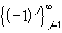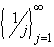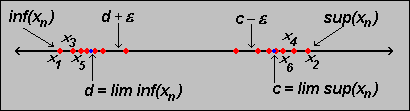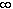#Interactive Real Analysis

## 3. Sequences of Numbers

### 3.4. Lim Sup and Lim Inf

When dealing with sequences there are two choices:

• the sequence converges
• the sequence diverges
While we know how to deal with convergent sequences, we don't know much about divergent sequences. One possibility is to try and extract a convergent subsequence, as described in the last section. In particular, Bolzano-Weierstrass' theorem can be useful in case the original sequence was bounded. However, we often would like to discuss the limit of a sequence without having to spend much time on investigating convergence, or thinking about which subsequence to extract. Therefore, we need to broaden our concept of limits to allow for the possibility of divergent sequences.
 Definition 3.4.1: Lim Sup and Lim Inf Letbe a sequence of real numbers. Define Aj = inf{aj , aj + 1 , aj + 2 , ...} and let c = lim (Aj). Then c is called the limit inferior of the sequence. Letbe a sequence of real numbers. Define Bj = sup{aj , aj + 1 , aj + 2 , ...} and let c = lim (Bj). Then c is called the limit superior of the sequence. In short, we have: lim inf(aj) = lim(Aj) , where Aj = inf{aj , aj + 1 , aj + 2 , ...} lim sup(aj) = lim(Bj) , where Bj = sup{aj , aj + 1 , aj + 2 , ...}
When trying to find lim sup and lim inf for a given sequence, it is best to find the first few Aj's or Bj's, respectively, and then to determine the limit of those. If you try to guess the answer quickly, you might get confused between an ordinary supremum and the lim sup, or the regular infimum and the lim inf.
 Examples 3.4.2:What is inf, sup, lim inf and lim sup for?What is inf, sup, lim inf and lim sup for?What is inf, sup, lim inf and lim sup forWhile these limits are often somewhat counter-intuitive, they have one very useful property:
 Proposition 3.4.3: Lim inf and Lim sup exist lim sup and lim inf always exist (possibly infinite) for any sequence of real numbers.

It is important to try to develop a more intuitive understanding about lim sup and lim inf. The next results will attempt to make these concepts somewhat more clear.

 Proposition 3.4.4: Characterizing lim sup and lim inf Letbe an arbitrary sequence and let c = lim sup(aj) and d = lim inf(aj). Then there is a subsequence converging to c there is a subsequence converging to d dlim inflim supc for any subsequence {} If c and d are both finite, then: given any> 0 there are arbitrary large j such that aj > c -and arbitrary large k such that ak < d +A little bit more colloquial, we could say:

• Aj picks out the greatest lower bound for the truncated sequences {aj}. Therefore Aj tends to the smallest possible limit of any convergent subsequence.
• Similarly, Bj picks the smallest upper bound of the truncated sequences, and hence tends to the greatest possible limit of any convergent subsequence.
Compare this with a similar statement about supremum and infimum.
 Example 3.4.5Ifis the sequence of all rational numbers in the interval [0, 1], enumerated in any way, find the lim sup and lim inf of that sequence.

The final statement relates lim sup and lim inf with our usual concept of limit.

 Proposition 3.4.6: Lim sup, lim inf, and limit If a sequence {aj} converges then lim sup aj = lim inf aj = lim aj Conversely, if lim sup aj = lim inf aj are both finite then {aj} converges.

To see that even simple concepts like lim inf and lim sup can result in interesting math consider the following unproven conjecture:

If pn is the n-th prime number, then lim inf pn+1 - pn = 2 and lim sup pn+1 - pn =The first equation is a conjecture, not yet proven, called the twin prime conjecture. In fact, it is not even known if the lim inf is finite. On the other hand, the second equation involving lim sup is known to be infinite because of arbitrary spaces between two primes.